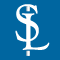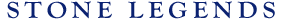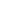HOMEPRODUCTSIDEA CENTERSERVICESTECHNICALABOUT USHello Guest Login  |  Become Member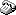800-398-1199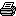214-398-1293MapEmail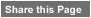Click to Share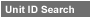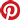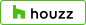Select which variables you would like to search by.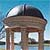Type DescriptionPrimary ViewPrimary ModifierUnit NameView Definitions

View Definitions

View DefinitionsX-Dim Y-Dim Z-Dim XX-Dim YY-Dim ZZ-Dim R-Dim D-Dim P-Dim
 Click column heading to re-sort results list.Product Name Primary View Modifier Pri Dimx  y  z Alt Dimxx  yy  zz Alt2 Dimr  d  p E\$timated Thumbnail Colossal Winds1 Gazebo Circular Column X = 155-1/2" Y = 120"Z = 155-1/2" XX = n/a YY = 144"ZZ = n/a R = n/a D = 155-1/2"P = n/a \$ 13756.63Grecian Temple Gazebo Circular X = 132" Y = 132"Z = 132" XX = n/a YY = n/aZZ = n/a R = 68-3/4" D = n/aP = n/a \$ 9446.89Kendal Verona Gazebo Octagonal X = 195" Y = 195"Z = n/a XX = n/a YY = n/aZZ = n/a R = n/a D = n/aP = n/a \$ 7378.00Lichfield Bellevue Gazebo Circular Balustrade X = 94" Y = 94"Z = 21-1/2" XX = n/a YY = n/aZZ = n/a R = 94" D = n/aP = n/a \$ 18028.00Oneida Temple Gazebo Circular Column X = 88" Y = 110"Z = 88" XX = n/a YY = n/aZZ = n/a R = n/a D = 88"P = n/a \$ 4669.96Renaissance Temple Gazebo Circular X = 137" Y = 108"Z = 137" XX = n/a YY = n/aZZ = n/a R = n/a D = 137"P = n/a \$ 6793.06